Question

# A projectile is fired with an initial speed of 37.4 m/s at an angle of 45.0...

A projectile is fired with an initial speed of 37.4 m/s at an angle of 45.0 ° above the horizontal on a long flat firing range.

Part A

Determine the maximum height reached by the projectile.

Express your answer using three significant figures and include the appropriate units.

Part B

Determine the total time in the air

Part C

Determine the total horizontal distance covered (that is, the range).

Given launch velocity,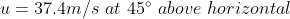Part A) Max height

Use formula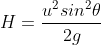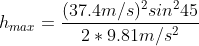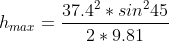ANSWER: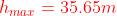Part B) Time of flight

Use formula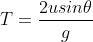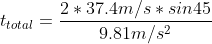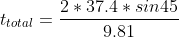ANSWER :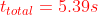====================

Part C) Range

Use formula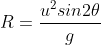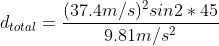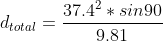ANSWER: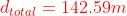===========================

Part D)

Given launch velocity,Vertical launch velocity,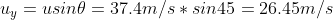Vertical velocity after 1.30s

Use formula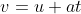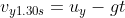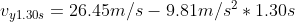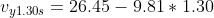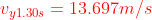Horizontal launch velocity,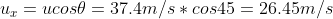Horizontal velocity after 1.30s

There is no acceleration in horizontal direction ,so horizontal velocity remains same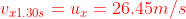Velocity after 1.30s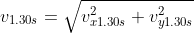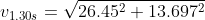Part D) ANSWER: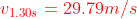#### Earn Coins

Coins can be redeemed for fabulous gifts.

Similar Homework Help Questions
• ### A projectile is fired with an initial speed of 20. m/s at an angle of 42.2°...

A projectile is fired with an initial speed of 20. m/s at an angle of 42.2° above the horizontal on a long flat firing range. Determine, a) the maximum height reached by the projectile, b) the total time in the air, c) the total horizontal distance covered (that is, the range), d) the speed of the projectile 1.50 s after firing. e) direction of the speed at 1.50 s.

• ### ASSIGNMENT PROBLEMS 1: A projectile is fired with an initial speed of 60.0 m/s at an...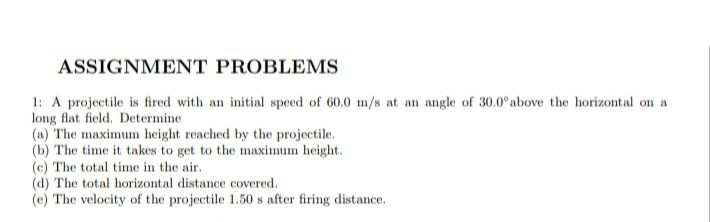ASSIGNMENT PROBLEMS 1: A projectile is fired with an initial speed of 60.0 m/s at an angle of 30.0°above the horizontal on a long flat field. Determine (a) The maximum height reached by the projectile. (b) The time it takes to get to the maximum height. (c) The total time in the air. (d) The total horizontal distance covered. (e) The velocity of the projectile 1.50 s after firing distance.

• ### A projectile is fired with Aprojectile is fired with an initial speed of 37.9 m/s at...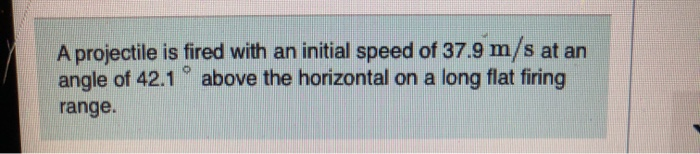A projectile is fired with Aprojectile is fired with an initial speed of 37.9 m/s at an angle of 42.1 above the horizontal on a long flat firing range. Part B Determine the total time in the air. Express your answer using three significant figures and include the appropriate units. HA ? trotal 5.081 Submit Previous Answers Request Answer xIncorrect; Try Again; 8 attempts remaining Review your calculations; you may have made a rounding error or used the wrong number...

• ### A projectile is fired with an initial speed of 36.2m/s at an angle of 41.1 degrees...

A projectile is fired with an initial speed of 36.2m/s at an angle of 41.1 degrees above the horizontal on a long flat firing range. determine maxiumum height reached by projectile total time in the air determine the range speed of the projectile 1.80s after firing

• ### Determine the total time in the ar Constants A projectile is fired with an initial speed...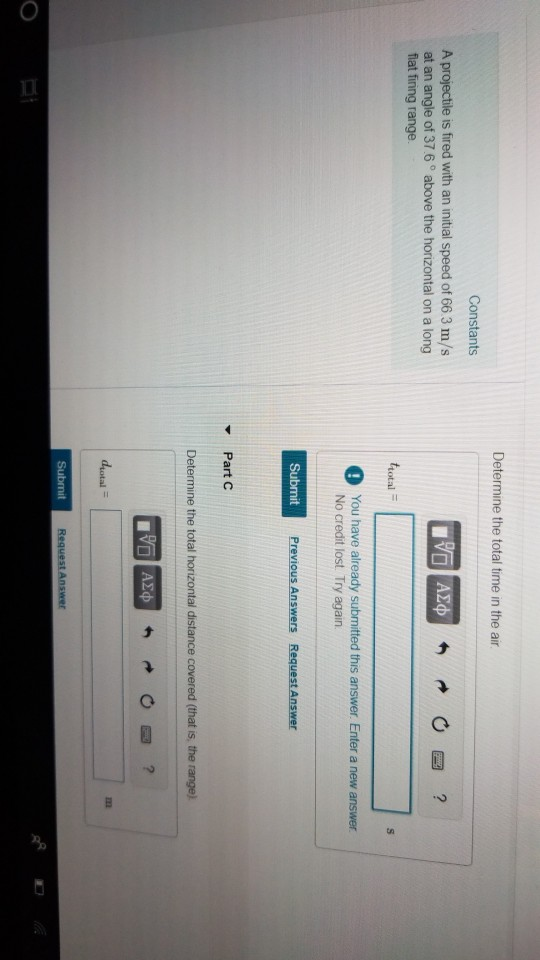Determine the total time in the ar Constants A projectile is fired with an initial speed of 66 3 m/s at an angle of 37 6 ° above the horizontal on a long total O You have already submitted this answer. Enter a new answer No credit lost. Try again Submit ▼ Part C Determine the total horizontal distance covered (that is, the range) dyotal Constants Part D A projectile is fired with an initial speed of 66.3 m/s at...

• ### A projectile is fired with an initial speed of 62.2 m/s at an angle of 43.4...

A projectile is fired with an initial speed of 62.2 m/s at an angle of 43.4 ∘ above the horizontal on a long flat firing range.Determine the direction of the projectile 1.32 s after firing.

• ### A projectile is fired with an initial speed of 250 m/s and angle of elevation 60°.

A projectile is fired with an initial speed of 250 m/s and angle of elevation 60°. The projectile is fired from a position 100 m above the ground. (Recall g ≈ 9.8 m/s2. Round your answers to the nearest whole number.) (a) Find the range of the projectile. (b) Find the maximum height reached. (c) Find the speed at impact.

• ### A projectile is fired with an initial speed of 250 m/s and angle of elevation 60°

A projectile is fired with an initial speed of 250 m/s and angle of elevation 60°. (Recall g ≈ 9.8 m/s2. Round your answers to the nearest whole number.) (a) Find the range of the projectile. (b) Find the maximum height reached. (c) Find the speed at impact.

• ### A projectile is fired with an initial speed of 190 m/s and angle of elevation 60°....

A projectile is fired with an initial speed of 190 m/s and angle of elevation 60°. The projectile is fired from a position 110 m above the ground. (Recall g ≈ 9.8 m/s2. Round your answers to the nearest whole number.) (sorry My bad) (a) Find the range of the projectile.   m (b) Find the maximum height reached.   m (c) Find the speed at impact. m/s